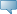# Lebanese American University WWW Information System

## Catalog Entries

Date
Sep 22, 2023Select the Course Number to get further detail on the course. Select the desired Schedule Type to find available classes for the course.

 MTH 305 - Probability and Statistics This course covers essentially the distribution theory, estimation and tests of statistical hypotheses. More specifically, the topics of this course include: Random variables, discrete probability, conditional probability, independence, expectation, standard discrete and continuous distributions, regression and correlation, point and interval estimation. Also included are illustrations from various fields. Course Learning Outcomes: 1)Students shall be able to use various counting principles to calculate simple probabilities. 2)Students shall be able to identify and use probability distributions of (discrete and continuous) random variables. 3)Students shall be able to use different statistics techniques including sampling distributions, sample estimation problems, sample tests of hypotheses, and simple linear regression and correlation. 3.000 Credit hours 3.000 Lecture hours Levels: Undergraduate Schedule Types: Lecture, Tutorial Computer Science & Mathematics Department